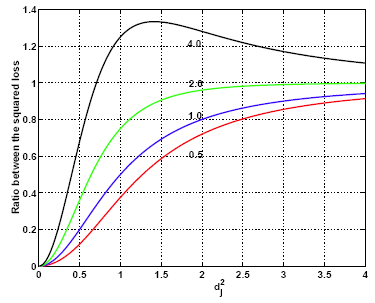# 5.2 - Compare Squared Loss for Ridge RegressionPrinter-friendly version

Let's compare squared loss with and without ridge regression, i.e.,  $E(\beta_j - \hat{\beta}_j)^2$.

Without shrinkage we know that $\hat{\beta}_j$ has a mean of $\beta_j$ since $\hat{\beta}_j$ is actually an unbiased estimator. Therefore, the expectation of the squared difference between $\beta_j$ and $\hat{\beta}_j$ is simply the variance of $\hat{\beta}_j$, which is given by  $\sigma^2 /d^{2}_{j}$ .

But, if we use shrinkage the estimates are no longer unbiased. Therefore, this expectation becomes the Bias2 + Variance of the ridge regression coefficient:

$\left(\beta_j -\beta_j \cdot\frac{d_{j}^{2}}{d_{j}^{2}+\lambda} \right)^2 +\frac{\sigma^2}{d_{j}^{2}} \cdot \left(\frac{d_{j}^{2}}{d_{j}^{2}+\lambda} \right)^2 = \frac{\sigma^2}{d_{j}^{2}} \cdot \frac{d_{j}^{2}\left(d_{j}^{2}+\lambda^2\frac{\beta_{j}^{2}}{\sigma^2} \right)}{(d_{j}^{2}+\lambda)^2}$

You can see that after all of this calculation the ratio of expected squared loss between linear regression and ridge regression is given by this factor:

$\frac{d_{j}^{2}\left(d_{j}^{2}+\lambda^2\frac{\beta_{j}^{2}}{\sigma^2} \right)}{(d_{j}^{2}+\lambda)^2}$.

If this factor is more than one, this means that ridge regression gives, on average, more squared loss as compared to linear regression. In other words, if this factor is greater than one then ridge regression is not doing a good job.

This factor depends on a lot of things. It depends on $\lambda$, $d_j$ , and the ratio $\beta_{j}^{2}/\sigma^2$.

Here is a plot where $\lambda$ is fixed at 1. The ratio between $\beta^2$ and $\sigma^2$ is set on the specific values, 0.5, 1.0, 2.0 and 4.0. Then we plotted the squared loss ratio on the y axis and the x axis is $d^{2}_{j}$ .Here you can see that when $\beta^2/\sigma^2$ is set at 0.5, 1.0 and 2.0 the squared loss ratio is always < 1 no matter what  $d^{2}_{j}$ is and you will always benefit by doing ridge regression (with $\lambda = 1$). If $\beta^2/\sigma^2$ becomes too big, at first the squared loss ratio is < 1 and you still benefit for small  $d^{2}_{j}$ . However, as $d^{2}_{j}$ gets big this ratio very quickly overshoots 1 and ridge regression is not doing as well as basic linear regression. The point of this graphic is to show you that ridge regression can reduce the expected squared loss even though it uses a biased estimator.